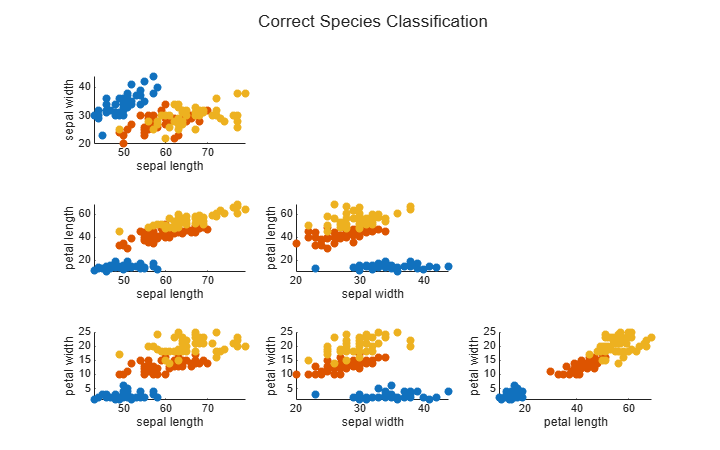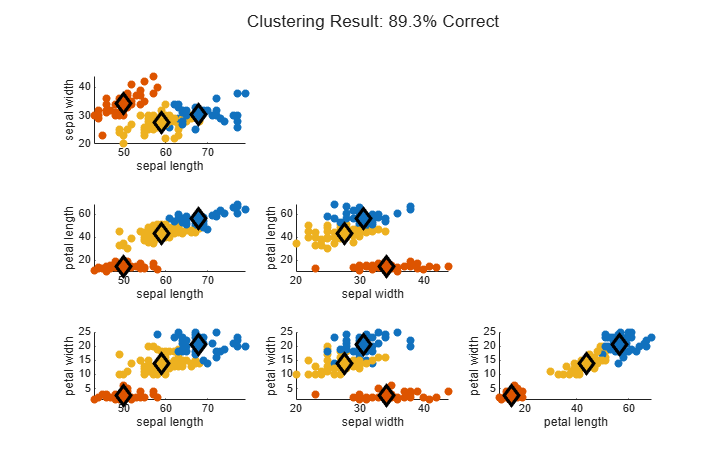# Fuzzy C-Means Clustering for Iris Data

This example shows how to use fuzzy c-means clustering for the iris data set. This dataset was collected by botanist Edgar Anderson and contains random samples of flowers belonging to three species of iris flowers: setosa, versicolor, and virginica. For each of the species, the data set contains 50 observations for sepal length, sepal width, petal length, and petal width.

Load the data set from the `iris.dat` data file.

`load iris.dat`

Partition the data into three groups named `setosa`, `versicolor`, and `virginica`.

```setosaIndex = iris(:,5)==1; versicolorIndex = iris(:,5)==2; virginicaIndex = iris(:,5)==3; setosa = iris(setosaIndex,:); versicolor = iris(versicolorIndex,:); virginica = iris(virginicaIndex,:);```

### Plot Data in 2-D

The iris data contains four dimensions representing sepal length, sepal width, petal length, and petal width. Plot the data points for each combination of two dimensions.

```Characteristics = {'sepal length','sepal width','petal length','petal width'}; pairs = [1 2; 1 3; 1 4; 2 3; 2 4; 3 4]; for i = 1:6 x = pairs(i,1); y = pairs(i,2); subplot(2,3,i) plot([setosa(:,x) versicolor(:,x) virginica(:,x)],... [setosa(:,y) versicolor(:,y) virginica(:,y)], '.') xlabel(Characteristics{x}) ylabel(Characteristics{y}) end```### Setup Parameters

Specify the options for clustering the data using fuzzy c-means clustering. These options are:

• `Nc` — Number of clusters

• M — Fuzzy partition matrix exponent, which indicates the degree of fuzzy overlap between clusters. For more information, see Adjust Fuzzy Overlap in Fuzzy C-Means Clustering.

• `maxIter` — Maximum number of iterations. The clustering process stops after this number of iterations.

• `minImprove` — Minimum improvement. The clustering process stops when the objective function improvement between two consecutive iterations is less than this value.

```Nc = 3; M = 2.0; maxIter = 100; minImprove = 1e-6;```

For more information about these options and the fuzzy c-means algorithm, see `fcm`.

### Compute Clusters

Fuzzy c-means clustering is an iterative process. Initially, the `fcm` function generates a random fuzzy partition matrix. This matrix indicates the degree of membership of each data point in each cluster.

In each clustering iteration, `fcm` calculates the cluster centers and updates the fuzzy partition matrix using the calculated center locations. It then computes the objective function value.

Cluster the data, displaying the objective function value after each iteration.

```clusteringOptions = [M maxIter minImprove true]; [centers,U] = fcm(iris,Nc,clusteringOptions);```
```Iteration count = 1, obj. fcn = 28838.424340 Iteration count = 2, obj. fcn = 21010.880067 Iteration count = 3, obj. fcn = 15272.280943 Iteration count = 4, obj. fcn = 11029.756194 Iteration count = 5, obj. fcn = 10550.015503 Iteration count = 6, obj. fcn = 10301.776800 Iteration count = 7, obj. fcn = 9283.793786 Iteration count = 8, obj. fcn = 7344.379868 Iteration count = 9, obj. fcn = 6575.117093 Iteration count = 10, obj. fcn = 6295.215539 Iteration count = 11, obj. fcn = 6167.772051 Iteration count = 12, obj. fcn = 6107.998500 Iteration count = 13, obj. fcn = 6080.461019 Iteration count = 14, obj. fcn = 6068.116247 Iteration count = 15, obj. fcn = 6062.713326 Iteration count = 16, obj. fcn = 6060.390433 Iteration count = 17, obj. fcn = 6059.403978 Iteration count = 18, obj. fcn = 6058.988494 Iteration count = 19, obj. fcn = 6058.814438 Iteration count = 20, obj. fcn = 6058.741777 Iteration count = 21, obj. fcn = 6058.711512 Iteration count = 22, obj. fcn = 6058.698925 Iteration count = 23, obj. fcn = 6058.693695 Iteration count = 24, obj. fcn = 6058.691523 Iteration count = 25, obj. fcn = 6058.690622 Iteration count = 26, obj. fcn = 6058.690247 Iteration count = 27, obj. fcn = 6058.690092 Iteration count = 28, obj. fcn = 6058.690028 Iteration count = 29, obj. fcn = 6058.690001 Iteration count = 30, obj. fcn = 6058.689990 Iteration count = 31, obj. fcn = 6058.689985 Iteration count = 32, obj. fcn = 6058.689983 Iteration count = 33, obj. fcn = 6058.689983 ```

The clustering stops when the objective function improvement is below the specified minimum threshold.

Plot the computed cluster centers as bold numbers.

```for i = 1:6 subplot(2,3,i); for j = 1:Nc x = pairs(i,1); y = pairs(i,2); text(centers(j,x),centers(j,y),int2str(j),'FontWeight','bold'); end end```